## TIFR PHD CS & SS 2013

 Question 1
An infinite two-dimensional pattern is indicated below.The smallest closed figure made by the lines is called a unit triangle. Within every unit triangle, there is a mouse. At every vertex there is a laddoo.
What is the average number of laddoos per mouse?
 A 3 B 2 C 1 D 1/2 E 1/3
 Question 2
Consider the following two types of elections to determine which of two parties A and B forms the next government in the 2014 Indian elections. Assume for simplicity an Indian population of size 545545(= 545*1001). There are only two parties A and B and every citizen votes.
TYPE C: The country is divided into 545 constituencies and each constituency has 1001 voters. Elections are held for each constituency and a party is said to win a constituency if it receive a majority of the vote in that constituency. The party that wins the most constituencies forms the next government.
TYPE P: There are no constituencies in this model. Elections are held throughout the country and the party that wins the most votes (among 545545 voters) forms the government.
Which of the following is true?
 A If a party forms the govt. by election TYPE C winning at least two-thirds of the constituencies, then it will also form the govt. by election TYPE P. B If a party forms the govt. by election TYPE C, then it will also form the govt. by election TYPE P C If a party forms the govt. by election TYPE P, then it will also form the govt. by election TYPE C D All of the above E None of the above
 Question 3
Three candidates, Amar, Birendra and Chanchal stand for the local election. Opinion polls are conducted and show that fraction a of the voters prefer Amar to Birendra, fraction b prefer Birendra to Chanchal and fraction c prefer Chanchal to Amar. Which of the following is impossible?
 A (a, b, c) = (0.51, 0.51, 0.51); B (a, b, c) = (0.61, 0.71, 0.67); C (a, b, c) = (0.68, 0.68, 0.68); D (a, b, c) = (0.49, 0.49, 0.49); E None of the above.
 Question 4
A biased coin is tossed repeatedly. Assume that the outcomes of different tosses are independent and probability of heads is 2/3 in each toss. What is the probability of obtaining an even number of heads in 5 tosses, zero being treated as an even number?
 A 121/243 B 122/243 C 124/243 D 125/243 E 128/243
 Question 5
The late painter Maqbool Fida Husain once coloured the surface of a huge hollow steel sphere, of radius 1 metre, using just two colours, red and blue. As was his style however, both the red and blue areas were a bunch of highly irregular disconnected regions. The late sculptor Ramkinkar Baij then tried to fit in a cube inside the sphere, the eight vertices of the cube touching only red coloured parts of the surface of the sphere. Assume π = 3.14 for solving this problem. Which of the following is true?
 A Baij is bound to succeed if the area of the red part is 10 sq. metres; B Baij is bound to fail if the area of the red part is 10 sq. metres; C Baij is bound to fail if the area of the red part is 11 sq. metres; D Baij is bound to succeed if the area of the red part is 11 sq. metres; E None of the above.
 Question 6
You are lost in the national park of Kabrastan. The park population consists of tourists and kabrastanis. Tourists comprise two-thirds of the population the park, and give a correct answer to requests for directions with probability 3/4. The air of Kabrastan has an amnesaic quality however, and so the answers to repeated questions to tourists are independent, even if the question and the person are the same. If you ask a Kabrastani for directions, the answer is always wrong.
Suppose you ask a randomly chosen passer-by whether the exit from the park is East or West. The answer is East. You then ask the same person again, and the reply is again East. What is the probability of East being correct?
 A 1/4 B 1/3 C 1/2 D 2/3 E 3/4
 Question 7
For any complex number z, arg z defines its phase, chosen to be in the interval 0 ≤ arg z < 360o. If z1, z2 and z3 are three complex numbers with the same modulus but different phases (arg z3 < arg z2 < arg z1 < 180o), then the quantityis a constant, and has the value
 A 2 B 1/3 C 1 D 3 E 1/2
 Question 8
Find the sum of the infinite series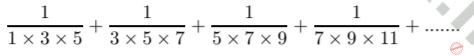A ∞ B 1/2 C 1/6 D 1/12 E 1/14
 Question 9
There are n kingdoms and 2n champions. Each kingdom gets 2 champions. The number of ways in which this can be done is:A a B b C c D d E e
 Question 10
Three men and three rakhsasas arrive together at a ferry crossing to find a boat with an oar, but no boatman. The boat can carry one or at the most two persons, for example, one man and one rakhsasa, and each man or rakhsasa can row. But if at any time, on any bank, (including those who maybe are in the boat as it touches the bank) rakhsasas outnumber men, the former will eat up the latter. If all have to go to the other side without any mishap, what is the minimum number of times that the boat must cross the river?
 A 7 B 9 C 11 D 13 E 15
 Question 11
Let there be a pack of 100 cards numbered 1 to 100. The ith card states: ”There are at most i − 1 true cards in this pack”. Then, how many cards of the pack contain TRUE statements?
 A 0 B 1 C 100 D 50 E None of the above
 Question 12
Among numbers 1 to 1000 how many are divisible by 3 or 7?
 A 333 B 142 C 475 D 428 E None of the above
 Question 13
Doctors A and B perform surgery on patients in stages III and IV of a disease. Doctor A has performed a 100 surgeries (on 80 stage III and 20 stage IV patients) and 80 out of her 100 patients have survived (78 stage III and 2 stage IV survivors). Doctor B has also performed 100 surgeries (on 50 stage III and 50 stage IV patients). Her success rate is 60/100 (49 stage III survivors and 11 stage IV survivors). A patient has been advised that she is equally likely to be suffering from stage III or stage IV of this disease.
Which doctor would you recommend to this patient and why?
 A Doctor A since she has a higher success rate B Doctor A since she specializes in stage III patients and the success of surgery in stage IV patients is anyway too low C Doctor B since she has performed more stage IV surgeries D Doctor B since she appears to be more successful E There is not enough data since the choice depends on the stage of the disease the patient is suffering from
 Question 14
An unbiased die is thrown n times. The probability that the product of numbers would be even is
 A 1/(2n) B 1/[(6n)!] C 1 − 6−n D 6−n E None of the above
 Question 15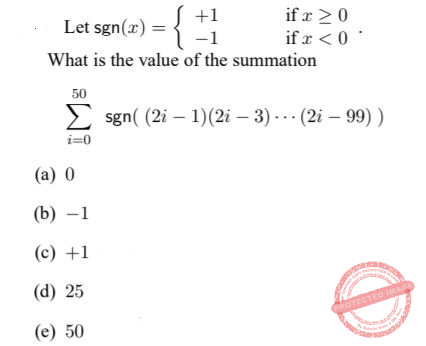A a B b C c D d E e
 Question 16
The minimum of the function f(x) = x loge(x) over the interval [1/2,∞) is
 A 0 B -e C − loge(2)/2 D −1/e E None of the above
 Question 17
A stick of unit length is broken into two at a point chosen at random. Then, the larger part of the stick is further divided into two parts in the ratio 4:3. What is the probability that the three sticks that are left CANNOT form a triangle?
 A 1/4 B 1/3 C 5/6 D 1/2 E loge(2)/2.
 Question 18
Consider three independent uniformly distributed (taking values between 0 and 1) random variables. What is the probability that the middle of the three values (between the lowest and the highest value) lies between a and b where 0 ≤ a < b ≤ 1
 A 3(1 − b)a(b − a) B 3((b − a) − (b2 − a2)/2) C 6(1 − b)a(b − a) D (1 − b)a(b − a) E 6((b2 − a2)/2 − (b3 − a3)/3).
 Question 19A a B b C c D d E e
 Question 20
Consider a well functioning clock where the hour, minute and the seconds needles are exactly at zero. How much time later will the minutes needle be exactly one minute ahead (1/60 th of the circumference) of the hours needle and the seconds needle again exactly at zero?
Hint: When the desired event happens both the hour needle and the minute needle have moved an integer multiple of 1/60 th of the circumference.
 A 144 minutes B 66 minutes C 96 minutes D 72 minutes E 132 minutes
 Question 21
Let G = (V,E) be a simple undirected graph on n vertices. A colouring of G is an assignment of colours to each vertex such that endpoints of every edge are given different colours. Let χ(G) denote the chromatic number of G, i.e., the minimum number of colours needed for a valid colouring of G. A set B ⊆ V is an independent set if no pair of vertices in B is connected by an edge. Let a(G) be the number of vertices in a largest possible independent set in G. In the absence of any further information about G we can conclude
 A χ(G) ≥ a(G) B χ(G) ≤ a(G) C a(G) ≥ n/χ(G) D a(G) ≤ n/χ(G) E None of the above
 Question 22
Consider polynomials in a single variable x of degree d. Suppose d < n/2. For such a polynomial p(x), let Cp denote the n-tuple (p(i))1≤i≤n. For any two such distinct polynomials p, q, the number of coordinates where the tuples Cp, Cq differ is
 A at most d B at most n − d C ) between d and n − d D at least n − d E none of the above
 Question 23
How many 4 × 4 matrices with entries from {0, 1} have odd determinant?
Hint: Use modulo 2 arithmetic
 A 20160 B 32767 C 49152 D 57343 E 65520
 Question 24
A set S together with partial order << is called a well order if it has no infinite descending chains, i.e. there is no infinite sequence x1, x2,... of elements from S such that xi+1 << xi and xi+1 ≠ xi for all i. Consider the set of all words (finite sequences of letters a-z), denoted by W, in dictionary order
 A Between ”aa” and ”az” there are only 24 words B Between ”aa” and ”az” there are only 224 words C W is not a partial order D W is a partial order but not a well order E W is a well order
 Question 25
Given a weighted directed graph with n vertices where edge weights are integers (positive, zero, or negative), determining whether there are paths of arbitrarily large weight can be performed in time
 A O(n) B O(n · log(n)) but not O(n) C O(n1.5) but not O(n log n) D O(n3) but not O(n1.5) E O(2n) but not O(n3)
 Question 26A a B b C c D d E e
 Question 27
Which of the following is not implied by P = NP?
 A 3SAT can be solved in polynomial time B Halting problem can be solved in polynomial time C Factoring can be solved in polynomial time. D Graph isomorphism can be solved in polynomial time E Travelling salesman problem can be solved in polynomial time
 Question 28
Which one of the following languages over the alphabet {0, 1} is regular?
 A The language of balanced parentheses where 0, 1 are thought of as (,) respectively B The language of palindromes, i.e., bit strings x that read the same from left to right as well as right to left C L = {0m2: 3 ≤ m} D The Kleene closure L∗, where L is the language in (c) above E {0m1n | 1 ≤ m ≤ n}
 Question 29
Suppose n straight lines are drawn on a plane. When these lines are removed, the plane falls apart into several connected components called regions. A region R is said to be convex if it has the following property: whenever two points are in R, then the entire line segment joining them is in R. Suppose no two of the n lines are parallel. Which of the following is true?
 A O(n) regions are produced, and each region is convex B O(n2) regions are produced but they need not all be convex C O(n2) regions are produced, and each region is convex D O(n log n) regions are produced, but they need not all be convex E All regions are convex but there may be exponentially many of them
 Question 30A a B b C c D d E e
 Question 31
Which of the following statements is FALSE?
 A The intersection of a context free language with a regular language is context free B The intersection of two regular languages is regular C The intersection of two context free languages is context free D The intersection of a context free language and the complement of a regular language is context free E The intersection of a regular language and the complement of a regular language is regular.
 Question 32
It takes O(n) time to find the median in a list of n elements, which are not necessarily in sorted order while it takes only O(1) time to find the median in a list of n sorted elements. How much time does it take to find the median of 2n elements which are given as two lists of n sorted elements each?
 A O(1) B O(log n) but not O(1) C O(√n) but not O(log n) D O(n) but not O(√n) E O(n log n) but not O(n)
 Question 33
Given a binary tree of the following form and having n nodes, the height of the tree is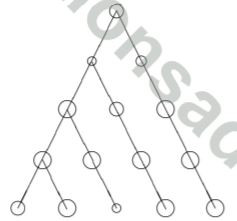A Θ(log n) B Θ(n) C Θ(√n) D Θ(n/ log n) E None of the above
 Question 34
Assume a demand paged memory system where ONLY THREE pages can reside in the memory at a time. The following sequence gives the order in which the program references the pages.
1, 3, 1, 3, 4, 2, 2, 4
Assume that least frequently used page is replaced when necessary. If there is more than one least frequently used pages then the least recently used page among them is replaced. During the program’s execution, how many times will the pages 1,2,3 and 4 be brought to the memory?
 A 2,2,2,2 times, respectively B 1,1,1,2 times, respectively C 1,1,1,1 times, respectively D 2,1,2,2 times, respectively E None of the above
 Question 35
Let G be an undirected graph with n vertices. For any subset S of vertices, the set of neighbours of S consists of the union of S and the set of vertices S that are connected to some vertex in S by an edge of G. The graph G has the nice property that every subset of vertices S of size at most n/2 has at least 1.5|S|-many neighbours. What is the length of a longest path in G?
 A O(1) B O(log log n) but not O(1) C O(log n) but not O(log log n) D O(√n) but not O(log n) E O(n) but not O(√n)
 Question 36A a B b C c D d E e
 Question 37
In a connected weighted graph with n vertices, all the edges have distinct positive integer weights. Then, the maximum number of minimum weight spanning trees in the graph is
 A 1 B n C equal to number of edges in the graph D equal to maximum weight of an edge of the graph E nn-2
 Question 38
Let S be a set of numbers. For x ∈ S, the rank of x is the number of elements in S that are less than or equal to x. The procedure Select(S, r) takes a set S of numbers and a rank r (1 ≤ r ≤ |S|) and returns the element in S of rank r. The procedure MultiSelect(S, R) takes a set of numbers S and a list of ranks R = {r1 < r2 < ··· < rk}, and returns the list {x1 < x2 < ··· < xk} of elements of S, such that the rank of xi is ri. Suppose there is an implementation for Select(S, r) that uses at most (constant · |S|) binary comparisons between elements of S. The minimum number of comparisons needed to implement MultiSelect(S, R) is
 A constant · |S| log |S| B constant · |S| C constant · |S||R| D constant · |R| log |S| E constant · |S|(1 + log |R|)
 Question 39
In a relational database there are three relations:
• Customers = C(CName),
• Shops = S(SName),
Which of the following relational algebra expressions returns the names of shops that have no customers at all? [Here Π is the projection operator.]
 A ΠSNameB B S − B C S − ΠSNameB D S − ΠSName((C × S) − B) E None of the above
 Question 40
Suppose n processors are connected in a linear array as shown below. Each processor has a number. The processors need to exchange numbers so that the numbers eventually appear in ascending order (the processor P1 should have the minimum value and the the processor Pn should have the maximum value).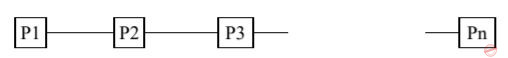The algorithm to be employed is the following. Odd numbered processors and even numbered processors are activated alternate steps; assume that in the first step all the even numbered processors are activated. When a processor is activated, the number it holds is compared with the number held by its right-hand neighbour (if one exists) and the smaller of the two numbers is retained by the activated processor and the bigger stored in its right-hand neighbour.
How long does it take for the processors to sort the values?
 A n log n steps B n2 steps C n steps D n1.5 steps E the algorithm is not guaranteed to sort
 Question 41
The unit step response of a discrete-time, linear, time-invariant system is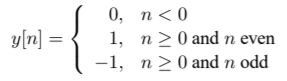Which of the following statements about the system is true?
 A the system is anti-causal B the system is memoryless C the system is bounded-input, bounded-output (BIBO) stable D there is not enough information to determine BIBO stability E none of the above
 Question 42
The output {y(n)} of a discrete time system with input {x(n)} is given byThe difference equation for the inverse system is given by
 A y(n) = x(n) − ax(n − 1) B ) y(n) − aN y(n − N) = x(n) − ax(n − 1) C If |a| < 1, then the answer is (a) above, otherwise the inverse does not exist D If |a| < 1, then the answer is (b) above, otherwise the inverse does not exist E None of the above
 Question 43
X and Y are jointly Gaussian random variables with zero mean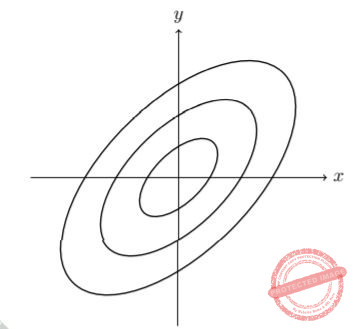A constant-pdf contour is where the joint density function takes on the same value. If the constant-pdf contours of X, Y are as shown above, which of the following could their covariance matrix K be: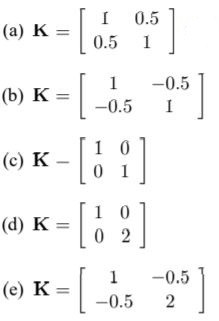A a B b C c D d E e
 Question 44
Consider a fair coin that has probability 1/2 of showing heads (H) in a toss and 1/2 of showing tails (T). Suppose we independently flip a fair coin over and over again. What is the probability that HT sequence occurs before TT?
 A 3/4 B 1/2 C 2/3 D 1/3 E 1/4
 Question 45
Let x(n) = sin(2πkn/N), n = 0, 1, ..., N − 1, where 2k ≠ N and 0 < k ≤ N − 1. Then the circular convolution of {x(n)} with itself is
 A N cos(4πkn/N) B N sin(4πkn/N) C −N cos(2πkn/N)/2 D −N sin(2πkn/N)/2 E None of the above
 Question 46
The two-dimensional Fourier transform of a function f(t, s) is given by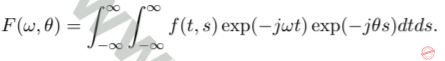Let δ(t) be the delta function and let u(t)=0 for t < 0 and u(t)=1 for t ≥ 0. If the Fourier transform is
F(ω, θ) = δ(ω − θ)/(jω + 1), then f(t, s) equals a constant multiple of
 A exp(−(t − s))u(t − s) B exp(−(t + s))u(t + s) C exp(−t)u(t)δ(s) D exp(−t)δ(t + s) E None of the above
 Question 47A a B b C c D d E e
 Question 48
The following circuit with an ideal operational amplifier isA A low pass filter B A high pass filter C A bandpass filter D A bandstop filter E An all pass amplifier
 Question 49
Let X and Y be two zero mean independent continuous random variables. Let Z1 = max(X, Y ), and Z2 = min(X, Y ). Then which of the following is TRUE
 A Z1 and Z2 are uncorrelated B Z1 and Z2 are independent C P(Z1 = Z2) = 1/2 . D Both (a) and (c) E Both (a) and (b)
 Question 50A a B b C c D d E e
 Question 51
Two matrices A and B are called similar if there exists another matrix S such that S−1AS = B.
Consider the statements:
(I) If A and B are similar then they have identical rank.
(I) If A and B are similar then they have identical trace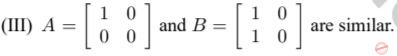Which of the following is TRUE.
 A Only I. B Only II. C Only III D Both I and II but not III. E All of I, II and III
 Question 52
Let A be a Hermitian matrix and let I be the Identity matrix with same dimensions as A. Then for a scalar α > 0, A + αI has
 A the same eigenvalues as of A but different eigenvectors B the same eigenvalues and eigenvectors as of A C the eigenvalues smaller than those of A and same eigenvectors as of A D eigenvalues and eigenvectors with no relation to those of A E None of the above
 Question 53
Let A be a square matrix and x be a vector whose dimensions match A. Let B† be the conjugate transpose of B. Then which of the following is not true:
 A x†A2x is always non-negative B x†Ax could be zero C x†Ax could be complex D If A = A† then x†Ax is real E If A = A† then x†Ay is complex for some vector y with same dimensions as x
 Question 54
X, Y, Z are integer valued random variables with the following two properties:
(i) X and Y are independent.
(ii) For all integer x, conditioned on the event {X = x}, we have that Y and Z are independent (in other words, conditioned on X, the random variables Y and Z are independent). Which of the following can we infer?
 A The random variables X and Z are independent B Conditioned on Y , the random variables X and Z are independent C Conditioned on Z, the random variables X and Y are independent D All of the above E None of the above
 Question 55A a B b C c D d E e
 Question 56
A surprise quiz contains three multiple choice questions; question 1 has 3 suggested answers, question 2 has four, and question 3 has two. A completely unprepared student decides to choose the answers at random. If X is the number of questions the student answers correctly, the expected number of correct answers is
 A 15/12 B 7/12 C 13/12 D 18/12 E None of the above
 Question 57
Consider four coins, three of which are fair, that is they have heads on one side and tails on the other and both are equally likely to occur in a toss. The fourth coin has heads on both sides. Given that one coin amongst the four is picked at random and is tossed, and the outcome is seen to be head, what is the probability that its other side is tails?
 A 1/2 B 3/8 C 3/5 D 3/4 E 5/7
 Question 58
Consider a coin tossing game between Santa and Banta. Both of them toss two coins sequentially, first Santa tosses a coin then Banta and so on. Santa tosses a fair coin: Probability of heads is 1/2 and probability of tails is 1/2. Banta’s coin probabilities depend on the outcome of Santa’s toss. If Santa sees an outcome heads then Banta gets a coin whose probability of heads is 3/4, and of tails is 1/4. If Santa tosses a tail then Banta gets a coin whose probability of tossing a tails is 3/4 and of heads is 1/4.
What is the probability of the event that they both have one head and one tail in the two trials conducted by each of them?
 A 1/2 B 5/16 C 3/16 D 1/4 E 1/3
 Question 59
Which of the following is true for polynomials defined over real numbers R.
 A Every odd degree polynomial has a real root. B Every odd degree polynomial has at least one complex root. C Every even degree polynomial has at least one complex root. D Every even degree polynomial has a real root. E None of the above
 Question 60
A function f : R → R is convex if for x, y ∈ R, α ∈ [0, 1], f(αx+ (1−α)y) ≤ αf(x)+ (1−α)f(y).
Which of the following is not convex:
 A x2 B x3 C x D x4 E ex
There are 60 questions to complete.